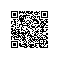# GPU编程(四): 并行规约优化

• 前言
• cuda-gdb
• 未优化并行规约
• 优化后并行规约
• 结果分析
• 最后

## 前言

• 之前第三篇也看到了, 并行方面GPU真的是无往不利, 现在再看下第二个例子, 并行规约. 通过这次的例子会发现, 需要了解GPU架构, 然后写出与之对应的算法的, 两者结合才能得到令人惊叹的结果.
• 这次也会简要介绍下cuda-gdb的用法, 其实和gdb用法几乎一样, 也就是多了个cuda命令.

## cuda-gdb

nvcc -g -G CUDAAdd.cu -o CUDAAdd.o#include <stdio.h>

__global__ void add(float * x, float *y, float * z, int n){
int index = threadIdx.x + blockIdx.x * blockDim.x;
int stride = blockDim.x * gridDim.x;

for (int i = index; i < n; i += stride){
z[i] = x[i] + y[i];
}
}

int main()
{
int N = 1 << 20;
int nBytes = N * sizeof(float);

float *x, *y, *z;
cudaMallocManaged((void**)&x, nBytes);
cudaMallocManaged((void**)&y, nBytes);
cudaMallocManaged((void**)&z, nBytes);

for (int i = 0; i < N; ++i)
{
x[i] = 10.0;
y[i] = 20.0;
}

dim3 blockSize(256);
// 4096
dim3 gridSize((N + blockSize.x - 1) / blockSize.x);

add << < gridSize, blockSize >> >(x, y, z, N);

cudaDeviceSynchronize();

float maxError = 0.0;
for (int i = 0; i < N; i++){
maxError = fmax(maxError, (float)(fabs(z[i] - 30.0)));
}
printf ("max default: %.4f\n", maxError);

cudaFree(x);
cudaFree(y);
cudaFree(z);

return 0;
}## 未优化并行规约#include <stdio.h>

const int    threadsPerBlock = 512;
const int    N        = 2048;
const int    blocksPerGrid    = (N + threadsPerBlock - 1) / threadsPerBlock; /* 4 */

__global__ void ReductionSum( float * d_a, float * d_partial_sum )
{
/* 申请共享内存, 存在于每个block中 */
__shared__ float partialSum[threadsPerBlock];

/* 确定索引 */
int    i    = threadIdx.x + blockIdx.x * blockDim.x;
int    tid    = threadIdx.x;

/* 传global memory数据到shared memory */
partialSum[tid] = d_a[i];

/* 传输同步 */
__syncthreads();

/* 在共享存储器中进行规约 */
for ( int stride = 1; stride < blockDim.x; stride *= 2 )
{
if ( tid % (2 * stride) == 0 )
partialSum[tid] += partialSum[tid + stride];
__syncthreads();
}

/* 将当前block的计算结果写回输出数组 */
if ( tid == 0 )
d_partial_sum[blockIdx.x] = partialSum;
}

int main()
{
int size = sizeof(float);

/* 分配显存空间 */
float    * d_a;
float    * d_partial_sum;

cudaMallocManaged( (void * *) &d_a, N * size );
cudaMallocManaged( (void * *) &d_partial_sum, blocksPerGrid * size );

for ( int i = 0; i < N; ++i )
d_a[i] = i;

/* 调用内核函数 */
ReductionSum << < blocksPerGrid, threadsPerBlock >> > (d_a, d_partial_sum);

cudaDeviceSynchronize();

/* 将部分和求和 */
int sum = 0;
for ( int i = 0; i < blocksPerGrid; ++i )
sum += d_partial_sum[i];

printf( "sum = %d\n", sum );

/* 释放显存空间 */
cudaFree( d_a );
cudaFree( d_partial_sum );

return(0);
}

## 优化后并行规约__global__ void ReductionSum( float * d_a, float * d_partial_sum )
{
// 相同, 略去
/* 在共享存储器中进行规约 */
for ( int stride = blockDim.x / 2; stride > 0; stride /= 2 )
{
if ( tid < stride )
partialSum[tid] += partialSum[tid + stride];
__syncthreads();
}
// 相同, 略去
}

### 结果分析

warp: GPU执行程序时的调度单位, 目前cuda的warp的大小为32, 同在一个warp的线程, 以不同数据资源执行相同的指令, 这就是所谓SIMT.## 最后使用钉钉扫一扫加入圈子
+ 订阅